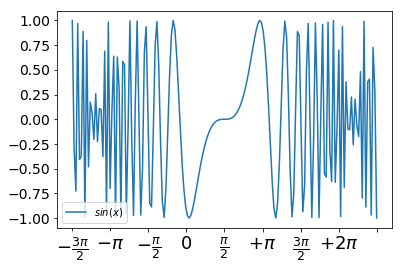## Matplotlib Tutorial: Spines and Ticks

### Moving the Border Lines and Polishing up the Axes NotationsThe word spine is most commonly known as the backbone or spinal cord of the human skeleton. Another meaning stands for a book's jacket. Our picture on the right side shows the spines of a cactus, artistically changed into something which looks like a ribcage. You will hardly find the usage of the word spine of matplotlib in a dictionary. Spines in matplotlib are the lines connecting the axis tick marks and noting the boundaries of the data area.

We will demonstrate in the following that the spines can be placed at arbitrary positions.

We will move around the spines in the course of this chapter so that the form a 'classical' coordinate syste. One where we have a x axis and a y axis and both go through the origin i.e. the point (0, 0)

We will show the naming of the spines in the following diagram: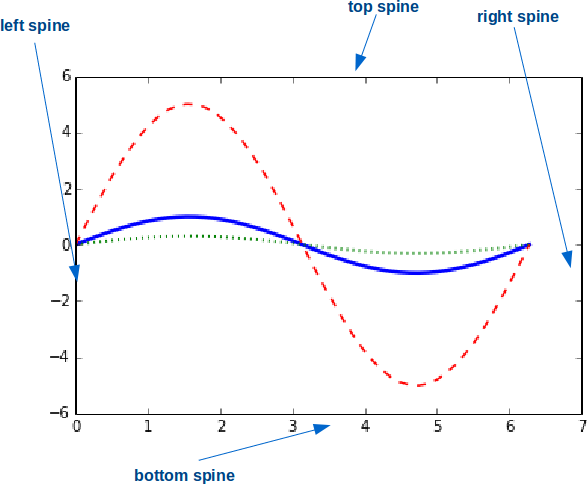We will move the spines to build a 'classical' coordinate system. To this purpose we turn the top and right spine invisible and move the bottom and left one around:

# the next "inline" statement is only needed,
# if you are working with "ipython notebook"
%matplotlib inline

import numpy as np
import matplotlib.pyplot as plt

X = np.linspace(-2 * np.pi, 2 * np.pi, 70, endpoint=True)
F1 = np.sin(2* X)
F2 = (2*X**5 + 4*X**4 - 4.8*X**3 + 1.2*X**2 + X + 1)*np.exp(-X**2)

fig, ax = plt.subplots()

# making the top and right spine invisible:
ax.spines['top'].set_color('none')
ax.spines['right'].set_color('none')

# moving bottom spine up to y=0 position:
ax.xaxis.set_ticks_position('bottom')
ax.spines['bottom'].set_position(('data',0))

# moving left spine to the right to position x == 0:
ax.yaxis.set_ticks_position('left')
ax.spines['left'].set_position(('data',0))

ax.plot(X, F1, X, F2)

plt.show()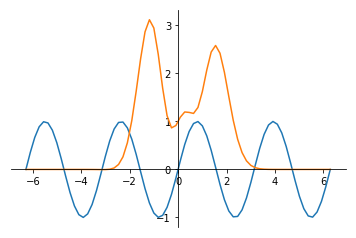### Customizing Ticks

Matplotlib has so far - in all our previous examples - automatically taken over the task of spacing points on the axis. We can see for example that the X axis in our previous example was numbered -6. -4, -2, 0, 2, 4, 6, whereas the Y axis was numbered -1.0, 0, 1.0, 2.0, 3.0

xticks is a method, which can be used to get or to set the current tick locations and the labels. The same is true for yticks:

import matplotlib.pyplot as plt
fig, ax = plt.subplots()

xticks = ax.get_xticks()
xticklabels = ax.get_xticklabels()
print(xticks, xticklabels)
for i in range(6):
print(xticklabels[i])

yticks = ax.get_yticks()
print(yticks)

[0.  0.2 0.4 0.6 0.8 1. ] <a list of 6 Text xticklabel objects>
Text(0, 0, '')
Text(0, 0, '')
Text(0, 0, '')
Text(0, 0, '')
Text(0, 0, '')
Text(0, 0, '')
[0.  0.2 0.4 0.6 0.8 1. ]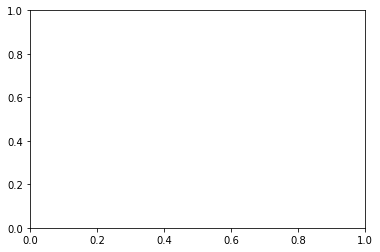As we said before, we can also use xticks to set the location of xticks:

import matplotlib.pyplot as plt
fig, ax = plt.subplots()

ax.set_xticks([7, 13, 19, 33, 42])

Output: :
[<matplotlib.axis.XTick at 0x7f8a1e5bd080>,
<matplotlib.axis.XTick at 0x7f8a1e5bdd68>,
<matplotlib.axis.XTick at 0x7f8a1e2237b8>,
<matplotlib.axis.XTick at 0x7f8a1e592a20>,
<matplotlib.axis.XTick at 0x7f8a1e5921d0>]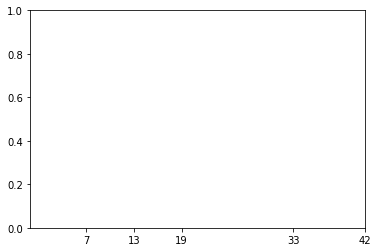Now, we will set both the locations and the labels of the xticks:

import matplotlib.pyplot as plt
fig, ax = plt.subplots()

ax.set_xticks([7, 13, 19, 33, 42])
ax.set_xticklabels(['Berlin', 'London', 'Hamburg', 'Toronto'])

Output: :
[Text(0, 0, 'Berlin'),
Text(0, 0, 'London'),
Text(0, 0, 'Hamburg'),
Text(0, 0, 'Toronto')]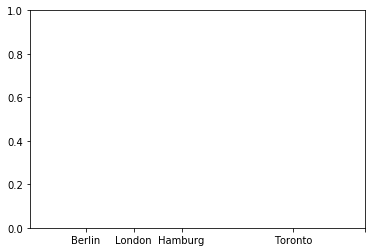Let's get back to our previous example with the trigonometric functions. Most people might consider factors of Pi to be more appropriate for the X axis than the integer labels:

import numpy as np
import matplotlib.pyplot as plt

X = np.linspace(-2 * np.pi, 2 * np.pi, 70, endpoint=True)
X = np.linspace(-2 * np.pi, 2 * np.pi, 70, endpoint=True)
F1 = np.sin(X**2)
F2 = X * np.sin(X)

fig, ax = plt.subplots()

# making the top and right spine invisible:
ax.spines['top'].set_color('none')
ax.spines['right'].set_color('none')
# moving bottom spine up to y=0 position:
ax.xaxis.set_ticks_position('bottom')
ax.spines['bottom'].set_position(('data',0))
# moving left spine to the right to position x == 0:
ax.yaxis.set_ticks_position('left')
ax.spines['left'].set_position(('data',0))

ax.set_xticks( [-6.28, -3.14, 3.14, 6.28])
ax.set_yticks([-3, -1, 0, +1, 3])
ax.plot(X, F1)
ax.plot(X, F2)

plt.show()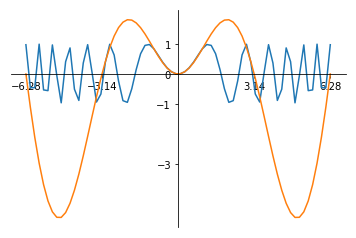There is an easier way to set the values of the xticks so that we do not have to caculate them manually. We use plt.MultipleLocator with np.pi/2 as argument:

import numpy as np
import matplotlib.pyplot as plt

X = np.linspace(-2 * np.pi, 2 * np.pi, 100)
F1 = np.sin(X)
F2 = 3 * np.sin(X)
fig, ax = plt.subplots()

ax.xaxis.set_major_locator(plt.MultipleLocator(np.pi / 2))

ax.plot(X, F1, X, F2)

plt.show()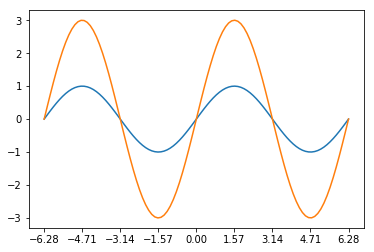### Setting Tick Labels

We want to rename the xticks now with custom markers. We will use the method xticks again for this purpose as we did in our previous examples. But this time we will call xticks with two parameters: The first one is the same list we used before, i.e. the positions on the x axis, where we want to have the ticks. The second parameter is a list of the same size with corresponding LaTeX tick marks, i.e. the text which we want to see instead of the values. The LaTeX notation has to be a raw string in most cases to suppress the escaping mechanism of Python, because the LaTeX notation heavily uses and relies on the backslash.

import numpy as np
import matplotlib.pyplot as plt

X = np.linspace(-2 * np.pi, 2 * np.pi, 100)
F1 = np.sin(X)
F2 = 3 * np.sin(X)
fig, ax = plt.subplots()

ax.xaxis.set_major_locator(plt.MultipleLocator(np.pi / 2))
ax.set_xticklabels([r'$-2\pi$', r'$-\frac{3\pi}{2}$', r'$-\pi$',
r'$-\frac{\pi}{2}$', 0, r'$\frac{\pi}{2}$',
r'$+\pi$', r'$\frac{3\pi}{2}$', r'$+2\pi$'])
ax.plot(X, F1, X, F2)

plt.show()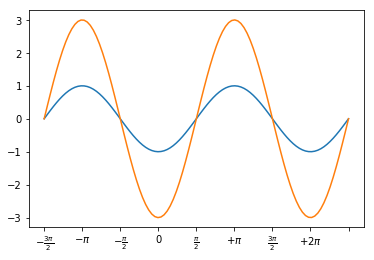We want to increase the legibility of the ticklabels. We will increase the font size, and we will render them on a semi transparant background.

print(ax.get_xticklabels())

<a list of 4 Text xticklabel objects>

for xtick in ax.get_xticklabels():
print(xtick)

Text(-6.28, 0, '$-2\\pi$')
Text(-3.14, 0, '$-\\pi$')
Text(3.14, 0, '$+\\pi$')
Text(6.28, 0, '$+2\\pi$')

labels = [xtick.get_text() for xtick in ax.get_xticklabels()]
print(labels)

['$-2\\pi$', '$-\\pi$', '$+\\pi$', '$+2\\pi$']


Let's increase the fontsize and make the font semi transparant:

import numpy as np
import matplotlib.pyplot as plt

X = np.linspace(-2 * np.pi, 2 * np.pi, 170, endpoint=True)
F1 = np.sin(X**3 / 2)

fig, ax = plt.subplots()

ax.xaxis.set_major_locator(plt.MultipleLocator(np.pi / 2))
ax.set_xticklabels([r'$-2\pi$', r'$-\frac{3\pi}{2}$', r'$-\pi$',
r'$-\frac{\pi}{2}$', 0, r'$\frac{\pi}{2}$',
r'$+\pi$', r'$\frac{3\pi}{2}$', r'$+2\pi$'])

for xtick in ax.get_xticklabels():
xtick.set_fontsize(18)
xtick.set_bbox(dict(facecolor='white', edgecolor='None', alpha=0.7 ))

for ytick in ax.get_yticklabels():
ytick.set_fontsize(14)
ytick.set_bbox(dict(facecolor='white', edgecolor='None', alpha=0.7 ))

ax.plot(X, F1, label="$sin(x)$")

ax.legend(loc='lower left')

plt.show()